## C# Average Method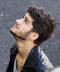Published on 14-Sep-2018 13:00:08
To find the average of integers in C#, use the Queryable Average() method.Let’s say the following is our integer array.var arr = new int[] { 10, 17, 25, 30, 40, 55, 60, 70 };Now, use the Average() method to get the average of the elements.double avg = Queryable.Average(arr.AsQueryable());Example Live Demousing System; ... Read More

## Implicit conversion from 32-bit unsigned integer (UInt) to Decimal in C#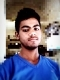Published on 14-Sep-2018 12:59:47
Implicit conversion of a 32-bit unsigned integer (UInt) to a Decimal requires you to first declare a UInt.uint val = 342741539;Now to convert it to decimal, just assign the value.decimal dec; // implicit dec = val;Example Live Demousing System; public class Demo {    public static void Main() {     ... Read More

## Implicit conversion from Byte to Decimal in C#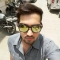Published on 14-Sep-2018 12:56:25
Byte represents an 8-bit unsigned integer.Implicit conversion of an 8-bit unsigned integer (Byte) to a Decimal is possible. Let us see how.Here’s our Byte value.byte val = 16;To implicitly convert, just assign the value as shown below −decimal dec; dec = val;Let us see the complete example.Example Live Demousing System; public ... Read More

## C# Program to convert a Double to an Integer Value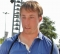Published on 14-Sep-2018 12:56:03
To convert a Double value to an integer value, use the Convert.ToInt32() method.Int32 represents a 32-bit signed integer.Let’s say the following is our double value.double val = 21.34;Now to convert it to Int32.int res = Convert.ToInt32(val);Let us see the complete example.Example Live Demousing System; public class Demo {    public static ... Read More

## C# Any Method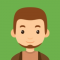Published on 14-Sep-2018 12:52:00
The Any method checks whether any of the element in a sequence satisfy a specific condition or not.If any element satisfy the condition, true is returned.Let us see an example.int[] arr = {5, 7, 10, 12, 15, 18, 20};Now, using Any() method, we will check whether any of the element ... Read More

## C# Program to convert a Double value to an Int64 valuePublished on 14-Sep-2018 12:51:29
To convert a Double value to an Int64 value, use the Convert.ToInt64() method.Int64 represents a 64-bit signed integer.Let’s say the following is our double value.double val = 23.951213e12;Now to convert it to Int64.long longVal = Convert.ToInt64(val);Let us see the complete example.Example Live Demousing System; public class Demo {    public static ... Read More

## C# Program to convert a Byte value to an Int32 value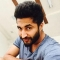Published on 14-Sep-2018 12:48:38
To convert a Byte value to an Int32 value, use the Convert.ToInt32() method.Int32 represents a 32-bit signed integer.Let’s say the following is our Byte value.byte val = Byte.MaxValue;;Now to convert it to Int32.int intVal = Convert.ToInt32(val);Let us see the complete example.Example Live Demousing System; public class Demo {    public static ... Read More

## C# Program to convert an Int32 value to a decimalPublished on 14-Sep-2018 12:48:06
To convert an Int32 value to a decimal, use the Convert.ToDecimal() method.Int32 represents a 32-bit signed integer.Let’s say the following is our Int32 value.int val = 2923;Now to convert it to decimal.decimal decVal = Convert.ToDecimal(val);Let us see the complete example.Example Live Demousing System; public class Demo {    public static void ... Read More

## ArgumentNullException in C#Published on 14-Sep-2018 12:45:49
The exception thrown when a null reference is passed to a method that does not accept it as a valid argument.Let us see an example.When we set a null parameter to int.Parse() method, then ArgumentNullException is thrown as shown below −Exampleusing System; class Demo {    static void Main() { ... Read More

## Get bounds of a C# three-dimensional arrayPublished on 14-Sep-2018 12:41:57
To get the bounds of a three-dimensional array, use the GetUpperBound() GetLowerBound() methods in C#.The parameter to be set under these methods is the dimensions i.e.Let’s say our array is −int[, , ] arr = new int[3, 4, 5];For a three-dimensional arrays, dimension 0.arr.GetUpperBound(0) arr.GetLowerBound(0)For a three-dimensional arrays, dimension 1.arr.GetUpperBound(1) ... Read More